NCERT Solutions for Class 7 Maths Chapter 14 Symmetry Ex 14.1 are part of NCERT Solutions for Class 7 Maths. Here we have given NCERT Solutions for Class 7 Maths Chapter 14 Symmetry Ex 14.1.

 Board CBSE Textbook NCERT Class Class 7 Subject Maths Chapter Chapter 14 Chapter Name Symmetry Exercise Ex 14.1 Number of Questions Solved 10 Category NCERT Solutions

## NCERT Solutions for Class 7 Maths Chapter 14 Symmetry Ex 14.1

Question 1.
Copy the figures with punched holes and find the axes of symmetry for the following :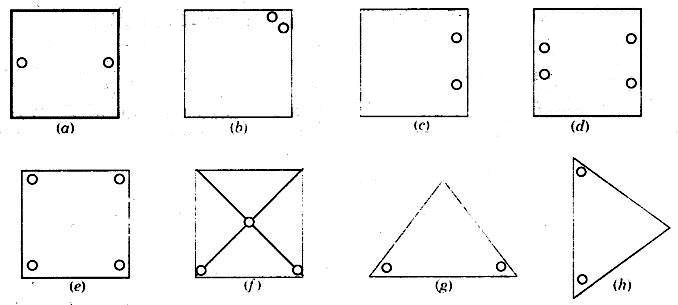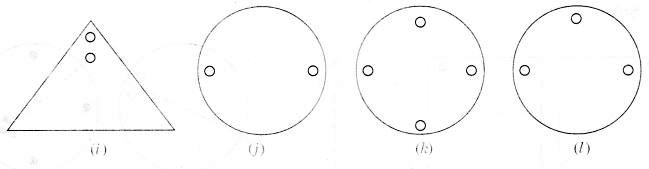Solution: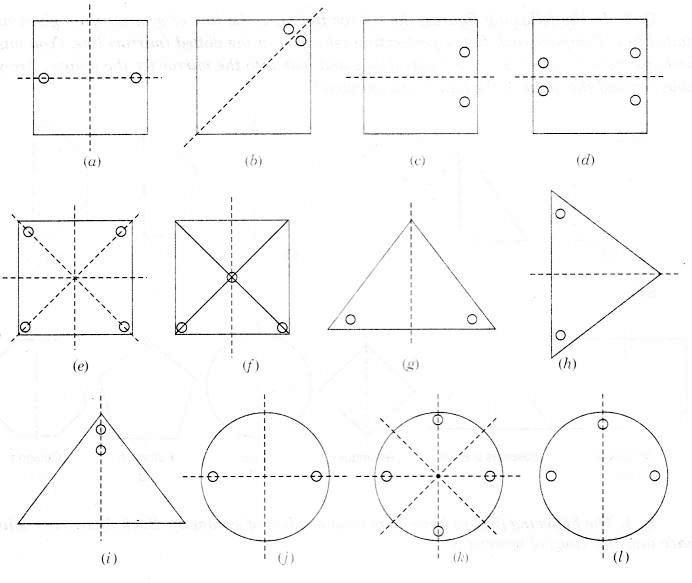Question 2.
Given the line(s) of symmetry, find the other hole(s):Solution: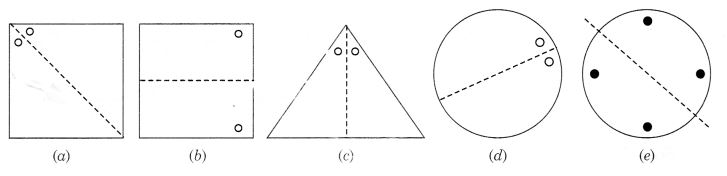Question 3.
In the following figures, the mirror line (i.e., the line of symmetry) is given as a dotted line. Complete each figure performing reflection in the dotted (mirror) line. (You might perhaps place a mirror along the dotted line and look into the mirror for the image). Are you able to recall the name of the figure you complete?
<Solution:Question 4.
The following figures have more than one line of symmetry. Such figures are said to have multiple lines of symmetry: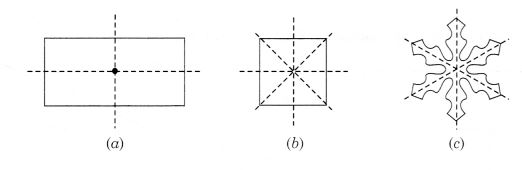Identify multiple lines of symmetry, if any, in each of the following figures: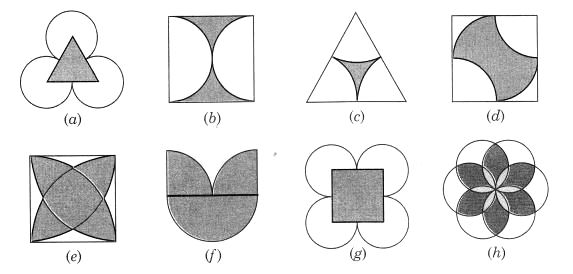Solution: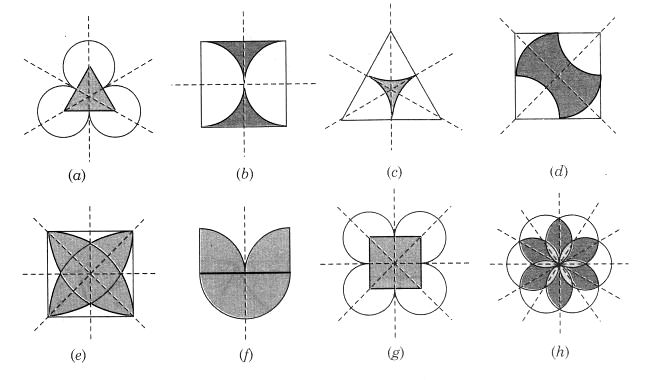Question 5.
Copy the figure given here. Take any one diagonal as a line of symmetry and shade a few more squares to make the figure symmetric about a diagonal. Is there more than one way to do that? Will the figure be symmetric about both the diagonals?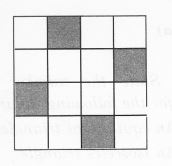Solution:Yes! there is more than one way to make the figure symmetric.

• Let us take the diagonal BD and shade the squares as shown in the figure to make the figure symmetric about BD.
• Similarly, the figure is symmetric about the diagonal AC. Thus, the figure is symmetric about both the diagonals.
• The figure is symmetric about EF and GH also.

Question 6.
Copy the diagram and complete each shape to be symmetric about the mirror line(s) :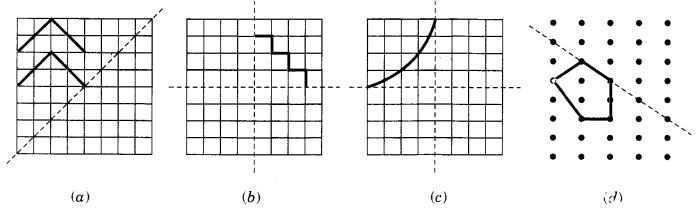Solution:Question 7.
State the number of lines of symmetry for the following figures :
(a) An equilateral triangle
(b) An isosceles triangle
(c) A scalene triangle
(d) A square
(e) A rectangle
(f) A rhombus
(g) A parallelogram
(i) A regular hexagon
(j) A circle.
Solution:Question 8.
What letters of the English alphabet have reflectional symmetry (i.e., symmetry related to mirror reflection) about
(a) a vertical mirror
(b) a horizontal mirror
(c) both horizontal and vertical mirrors.
Solution:Question 9.
Give three examples of shapes with no line of symmetry.
Solution: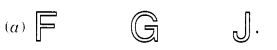(b) A scalene triangle,
(c) A parallelogram

Question 10.
What other name can you give to the line of symmetry of

1. an isosceles triangle?
2. a circle?

Solution:

1. Median
2. Diameter

We hope the NCERT Solutions for Class 7 Maths Chapter 14 Symmetry Ex 14.1 helps you. If you have any query regarding NCERT Solutions for Class 7 Maths Chapter 14 Symmetry Ex 14.1, drop a comment below and we will get back to you at the earliest.